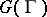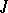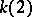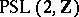Gamma-invariant in the theory of modular forms-invariant

Letbe a Fuchsian group of the first kind, acting on the real hyperbolic plane. Suppose thatis a group of genus zero, i.e. the number of hyperbolic generators ofis equal to zero. Letbe a conformal mapping of the fundamental domainofonto the complex planethat is extended as an automorphic function to the whole of. In other words, there exists an isomorphism of complex manifolds between the compactification ofand the Riemann sphere which induces an univalent automorphic functionon. The functionis defined up to a fractional-linear mapping and satisfies the Schwarz differential equation (see [a1]; see also Schwarz differential; Schwarz equation).

The functionis called a gamma- or automorphic (modular) invariant.

The first example of a gamma-invariant,, was discovered and investigated in the Abel–Gauss–Jacobi and Eisenstein–Weierstrass theory of elliptic and elliptic modular functions between 1820– 1850 (see [a2]). The functionwas obtained as a function of the quotient of the periods of a certain elliptic integral. It corresponds to the level-two congruence subgroup of the modular group. For the modular group itself, the invariantwas constructed in 1877 by R. Dedekind and, one year later and independently, by F. Klein. In this definition,is a certain rational function of the two main modular holomorphic Eisenstein seriesand. These two classical invariantsandsatisfy the modular equation.

Gamma-invariants play an important role in various domains of mathematics and mathematical physics. For example, in algebraic number theory the modular equation and its generalizations were used by Ch. Hermite and L. Kronecker to obtain the transcendental solution of the general algebraic equation of the fifth degree (see [a2]). The modular invariant is important in the extension of the Kronecker theorem on Abelian fields for imaginary quadratic ground fields and, more generally, in class field theory (see [a3], [a4]). Other important interpretations and developments of this theory are in algebraic geometry (the theory of elliptic and modular curves) and in the theory of Dirichlet-series (see [a5], [a6]; see also Dirichlet-function).

Automorphic invariants participate also in Selberg's theory of the trace formula for constructing resolvents of automorphic Laplacians (automorphic Green functions) (see [a1], [a6]).

In [a7] modular equations are used for investigating non-congruence subgroups of the modular group.

There is a mysterious relationship between simple finite groups (the Monster, cf. Simple finite group) and automorphic invariants (see [a8], [a9], [a10]). In particular, via this relation there is a connection between modular invariants and mathematical physics (string theory [a11], vertex operator algebra and quantum field theory [a12], [a13], [a14], [a15]).